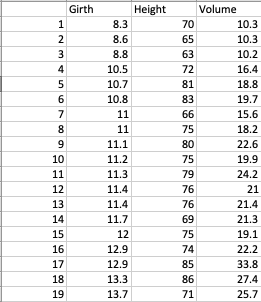# Sorting A Pandas.series

## Overview:

• Sorting is the process of ordering a sequence of objects, numbers, records and so on based on specific criteria.
• In Computer Science, the criteria for sorting is generally specified through a predicate function which tells, among two objects in question which one should be given precedence in the sorted order.
• The most common criteria applied for sorting is "Greater Than" and "Less Than" conditions. Based on these two criteria, objects are arranged in either increasing order or in decreasing order.

## Sorting the elements of a pandas.Series:

• The Python class pandas.Series implements a one-dimensional heterogeneous container with multitude of statistical and mathematical functions for Data Analysis.
• A Series in pandas can be sorted either based on the values it hold or its index.
• Also the Series elements can be arranged in either decreasing order or increasing order.
• While sorting instances of user defined classes it is recommended to implement the rich comparison methods __lt__(), __gt__(), __le__(), __ge__(), __eq__() or at least the __lt__(), __eq__() methods.
• The Python example code below loads a CSV file with data about Black Cherry Trees and selects height data into a pandas.Series instance.## Example - Sort a pandas.series based on the values:

 # Example Python program to sort the elements present in a pandas.Series import pandas as pds   # Path of the CSV file containing Girth, Height and Volume data of Black Cherry Trees csvPath = "./trees.csv"; dataFrame = pds.read_csv(csvPath);   # Select height of the Black Cherry Trees into a pandas.Series instance series = dataFrame["Height"]; sortedSeries = series.sort_values();   print("First ten values from the sorted series:"); print(sortedSeries.head(10));

## Output:

 2     63 19    64 1     65 6     66 13    69 0     70 18    71 3     72 23    72 22    74

## Example - Sort class objects stored in a pandas.Series:

This pandas example stores multiple class objects in a pandas.Series. The class Part implements the __lt__() method and the __eq__() method.The developer can choose to implement the the sorting either based on either member - id or price. This implementation uses the price to determine the sorting order.

 # Example Python program to sort class instances stored in a pandas.Series import pandas as pds   # Class definition of some part(of a car, furniture...) class Part:     def __init__(self, id, price):         self.id = id;         self.price = price;       # Providing a price centric sort     def __lt__(self, part):         return self.price < part.price;       # Providing a price centric sort     def __eq__(self, part):         return self.price == part.price;       # Describe the part...the string representation     def __str__(self):         return "Id:%d,Price:%.2f"%(self.id,self.price);   # Create parts        part1 = Part(1, 98.5); part2 = Part(2, 35.6); part3 = Part(3, 47.4); part4 = Part(4, 12.4); part5 = Part(5, 9.4);   # Store them in a series series = pds.Series([part1, part2, part3, part4, part5]);   # Sort the parts based on price partsSorted = series.sort_values();   # Print the parts in sorted order print("Parts sorted based on their price:") print(partsSorted);

## Output:

 Parts sorted based on their price: 4     Id:5,Price:9.40 3    Id:4,Price:12.40 1    Id:2,Price:35.60 2    Id:3,Price:47.40 0    Id:1,Price:98.50 dtype: object

## Example - Sorting a pandas.Series based on its index:

 # Example Python program to sort a pandas.Series # based on its index import pandas as pds   series = pds.Series(["e", "a", "i", "u", "o"], index=(1,0,2,4,3)); sorted = series.sort_index();   print("Unsorted:"); print(series);   print("Sorted:") print(sorted);

## Output:

 Unsorted: 1    e 0    a 2    i 4    u 3    o dtype: object Sorted: 0    a 1    e 2    i 3    o 4    u dtype: object